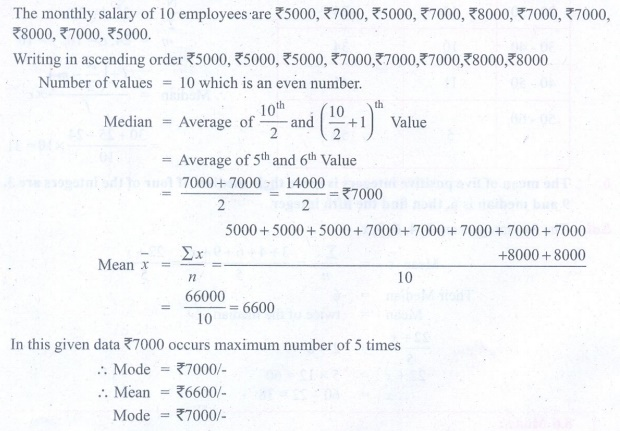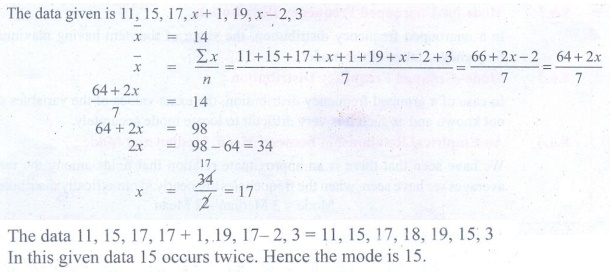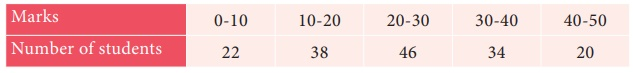Home | | Maths 9th std | Exercise 8.3: Statistics: Mode

# Exercise 8.3: Statistics: Mode

Maths : Statistics: Book Back, Exercise, Example Numerical Question with Answers, Solution: Exercise 8.3: Statistics: Mode

Statistics: Mode

Exercise 8.3

1. The monthly salary of 10 employees in a factory are given below : 5000,7000,5000,7000,8000,7000,7000,8000,7000,5000 Find the mean, median and mode.

Solution:2. Find the mode of the given data : 3.1, 3.2, 3.3, 2.1, 1.3, 3.3, 3.1

Solution:3. For the data 11, 15, 17, x+1, 19, x–2, 3 if the mean is 14 , find the value of x. Also find the mode of the data.

Solution:4. The demand of track suit of different sizes as obtained by a survey is given below:Which size is in greater demanded?

Solution:5. Find the mode of the following data:Solution:6. Find the mode of the following distribution:Solution:Tags : Numerical Problems with Answers, Solution | Statistics | Maths , 9th Maths : UNIT 8 : Statistics
Study Material, Lecturing Notes, Assignment, Reference, Wiki description explanation, brief detail
9th Maths : UNIT 8 : Statistics : Exercise 8.3: Statistics: Mode | Numerical Problems with Answers, Solution | Statistics | Maths